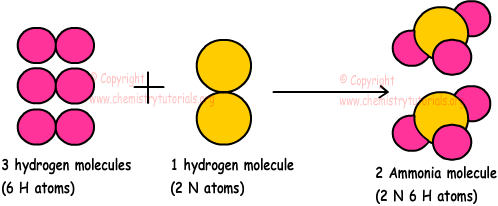## Chemical Reactions with Examples

Chemical Reactions with Examples

Changes in the chemical structures of the matters are called chemical changes. Atoms or molecules of matter interact with each other in these changes. In general, bonds, keeping atoms or molecules together, are broken and after chemical change, new bonds are produced between atoms or molecules. This means that after chemical change, new matters having different properties are formed. In physical and chemical changes, structure of nuclei does not change.

For example;

H2 +1/2O2→H2O

Hydrogen + Oxygen → Water

Hydrogen and oxygen lost their properties and they form water having totally different properties.

"Chemical reactions" are symbolization of chemical changes with element/compounds symbols, arrow and coefficients. In chemical reactions, reactants are written at left side of arrow and products are written at right side of arrow. Number of moles of reactants and products give us coefficient in the chemical reactions. Physical states of matters are also shown in the chemical reactions in brackets like "s" for solids, "g" for gases, "l" for liquids and "aq" for matters in solutions.

P4(s) + 5O2(g) + 6H2O(l) → 4H3PO4(l)

1mol P4 solid, 5moles O2 gas, 6moles H2O liquid react to produce 4moles H3PO4 liquid.

If phases of matters in the chemical reaction are same, we call them homogeneous reactions but if they are not same we call them heterogeneous reactions as given in the examples below.

2NH3(g) → N2(g) + 3H2(g) Homogeneous reaction

CH4(g) + 2O2(g) → CO2(g) + 2H2O(l) Heterogeneous reaction

Constant Values in Chemical Reactions

• Mass is always conserved in chemical reactions. In other words, total mass of reactants is equal to total mass of products.

Example:

2H2 + O2 → 2H2O

H:1 and O:16

Mass of reactants=2.(2.1)+(16.2)=36 g

Mass of products=2.(2.1+16)=36 g

• Number of atoms and kinds of atoms are conserved
• Structure of nuclei is conserved
• Total numbers of protons, neutrons and electrons are conserved
• In ionic chemical reactions, total charge is conserved

Example:

H+1 + OH- → H2O

+1+(-1)=0

Variables in Chemical Reactions

• Numbers and structure of electrons in atoms can change
• Volumes and radii of atoms can change
• Chemical bonds can change
• Total volume, number of moles and molecules can not be conserved

Example:

3H2 + N2 → 2NH3Number of moles of reactants =3+1=4moles

Number of moles of products=2moles

Number of moles is not conserved

• Physical and chemical properties of matters change

Example: Which ones of the following statements are true for following reaction;

3X2(g) + Y2(g) → 2X3Y(g)

I. Number of molecules decreases

II. Total mass increases

III. Total volume decreases

(Matters are placed in closed container)

3 moles X2 and 1mol Y2 are reactants, and 2 moles X3Y products, so 4 moles reactants become 2 mole products. Since number of moles decreases, number of molecules also decreases. Volume of container is constant thus, it does not change during and after reaction. Matter is conserved in all chemical reactions.

I is true and II and III are false.

Chemical Reactions Exams and  Problem Solutions

Related